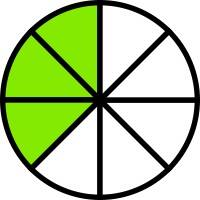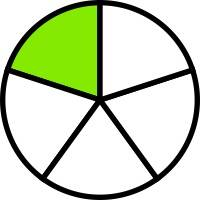# Which Is Bigger 1/5 Or 3/8?

Here is 3/8Here is 1/53/8 is larger.

6 People thanked the writer.
Virginia Lou commented
Very visual, very clear...
I prefer visual aids ... don't really like math! LOL :)
That would be 1/5 it is bigger
thanked the writer.
Simple math. Use a common denominater 5x8=40.....1/5 = 8/40    3/8=15/40 thus 3/8 is 7/40 larger than 1/5
1 Person thanked the writer.
Actually, 3/8 is really greater
2 People thanked the writer.

## 1/5 is bigger. I know it might be weird, since people are taught that the bigger number is the better value, but with fractions, the smaller the denominater, the bigger the piece is and the better chance it has of getting to a whole number. Do you understand? Hopefully this helps. : )

thanked the writer.
Mike J commented
Wrong.

First, divide 1 by 5, you get .20.

Now, divide 1 by 8 and multiply by 3, you get .375.

3/8 is greater.
1/5 is bigger:)
thanked the writer.
Mike J commented
No, 3/8 is bigger.
First, find a common denominator of 5 and 8 (40)
Next, convert 1/5 --> 8/40 and 3/8 ---> 15/40.
Finally, compare. 15/40 (3/8) is bigger than 8/40 (1/5)
Mike J commented
1/5 = 1 ÷ 5 = 0.200

3/8 = 3 ÷ 8 = 0.375

0.375 > 0.200
sam silverman commented
Wow incredibly arrogant of you!